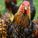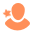## CMS DevelopmentContributeur de premier rang

## Metric to Imperial Calculation

SOLVE

I've have some data in HubDB that represents a metric measuremnt in mm for example 3174. A calculation then converts it to feet and inches;

``````{% set metric = (row.gf_kitchen_met_1) %}
{% set imperial = 304.8 %}
{% set calculation = (metric / imperial ) | round (1, 'floor') %}

Original = {{row["gf_kitchen_met_1"]}}<br>
Converted = {{ calculation }}"``````

The example above correctly shows  3174 converted to 10.4

Is there a way to show the converted number with the correct symbols, i.e. 10'4"  ?

1 Accepted solutionSolution
Contributeur de premier rang

## Metric to Imperial Calculation

SOLVE

I figured it out using the 'replace' function  😀 ...

``New calculation = {{ calculation|replace('.','&apos;') }}"``Contributeur de premier rang

## Metric to Imperial Calculation

SOLVE

Well sort of @dennisedson. I've had another look at the above and it's only caluclating the 'ft' not inches. Howver using the below is a lot more accurate...

``````{% for row in housetype_db %}
<!-- Convert mm to ft & inches -->
{% set inches = (row.gf_kitchen_met_1 / 25.4) %}
{% set feet = (inches / 12)|round(0, 'floor') %}
{% set measurement = (inches % 12)|round(0) %}
<!-- Run calculation -->
{% if measurement >= 12 %}
<p>{{ feet + 1 }}'0"</p>
{% else %}
<p>{{ feet }}'{{ measurement }}"</p>
{% endif %}
{% endfor %}``````Solution
Contributeur de premier rang

## Metric to Imperial Calculation

SOLVE

I figured it out using the 'replace' function  😀 ...

``New calculation = {{ calculation|replace('.','&apos;') }}"``Community Manager

## Metric to Imperial Calculation

SOLVE

Solved in 11 minutes from initial post to answering yourself. 🏍

Thanks,

DennisMake sure to subscribe to our YouTube channel where you can find the HubSpot Community Developer Show